Coin and die

Flip a coin and then roll a six-sided die. How many possible combinations are there?

Result

n =  12

Solution:Leave us a comment of this math problem and its solution (i.e. if it is still somewhat unclear...):Be the first to comment!To solve this verbal math problem are needed these knowledge from mathematics:

See also our variations calculator. Would you like to compute count of combinations?

Next similar math problems:

1. Theorem proveWe want to prove the sentence: If the natural number n is divisible by six, then n is divisible by three. From what assumption we started?
2. Lock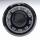Combination lock will open when the right choice of 5 numbers (from 1 to 12 inclusive) is selected. A. How many different lock combinations are possible? B. Is he combination lock named appropriately?
3. Pins 2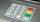how many different possible 4 digits pins can be found on the 10-digit keypad?
4. Friends in cinema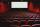5 friends went to the cinema. How many possible ways can sit in a row, if one of them wants to sit in the middle and the remaining's place does not matter?
5. Cars plates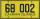How many different licence plates can country have, given that they use 3 letters followed by 3 digits?
6. Football league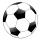In the 5th football league is 10 teams. How many ways can be filled first, second and third place?
7. MetalsIn the Hockey World Cup play eight teams, determine how many ways can they win gold, silver and bronze medals.
8. PIN - codesHow many five-digit PIN - code can we create using the even numbers?
9. Olympics metalsIn how many ways can be win six athletes medal positions in the Olympics? Metal color matters.
10. MedalsIn how many ways can be divided gold, silver and bronze medal among 21 contestant?
11. Flags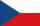How many different flags can be made from colors white, red, green, purple, orange, yellow, blue so that each flag consisted of three different colors?
12. Bits, bytes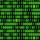Calculate how many different numbers can be encoded in 16-bit binary word?
13. Practice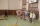How many ways can you place 20 pupils in a row when starting on practice?
14. Chess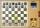How many different ways can initiate a game of chess (first pass)?
15. VariationsDetermine the number of items when the count of variations of fourth class without repeating is 42 times larger than the count of variations of third class without repetition.
16. Task of the year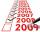Determine the number of integers from 1 to 106 with ending four digits 2006.
17. A three-digit numbers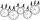Determine the total number of positive three-digit numbers that contain a digit 6.